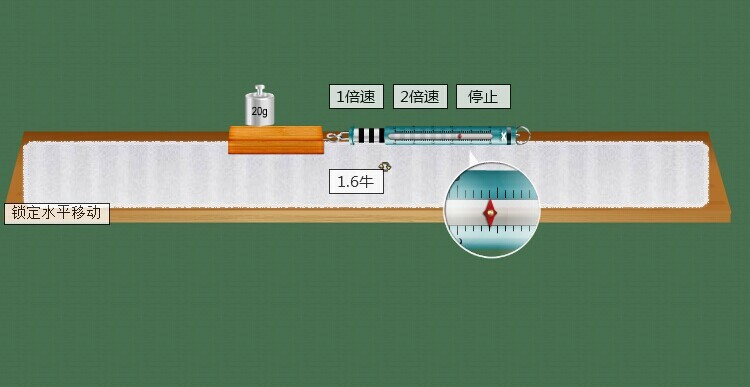### 高中物理公式大汇总(1)------直线运动

1)匀变速直线运动

1.平均速度V平=s/t(定义式)

2.有用推论Vt2-Vo2=2as

3.中间时刻速度Vt/2=V平=(Vt+Vo)/2

4.末速度Vt=Vo+at

5.中间位置速度Vs/2=[(Vo2+Vt2)/2]1/2

6.位移s=V平t=Vot+at2/2=Vt/2t

7.加速度a=(Vt-Vo)/t{以Vo为正方向，a与Vo同向(加速)a>0;反向则a<0｝

8.实验用推论Δs=aT2{Δs为连续相邻相等时间(T)内位移之差｝

9.主要物理量及单位：初速度(Vo)：m/s;加速度(a)：m/s2;末速度(Vt)：m/s;时间(t)秒(s);位移(s)：米(m);路程：米;速度单位换算：1m/s=3.6km/h。

注：

(1)平均速度是矢量;(2)物体速度大，加速度不一定大;(3)a=(Vt-Vo)/t只是量度式，不是决定式;

(4)其它相关内容：质点.位移和路程.参考系.时间与时刻;速度与速率.瞬时速度。

2)自由落体运动

1.初速度Vo=02.末速度Vt=gt3.下落高度h=gt2/2(从Vo位置向下计算)4.推论Vt2=2gh

注：

(1)自由落体运动是初速度为零的匀加速直线运动，遵循匀变速直线运动规律;

(2)a=g=9.8m/s2≈10m/s2(重力加速度在赤道附近较小，在高山处比平地小，方向竖直向下)。

(3)竖直上抛运动

1.位移s=Vot-gt2/22.末速度Vt=Vo-gt(g=9.8m/s2≈10m/s2)

3.有用推论Vt2-Vo2=-2gs4.上升最大高度Hm=Vo2/2g(抛出点算起)

5.往返时间t=2Vo/g(从抛出落回原位置的时间)

注：

(1)全过程处理：是匀减速直线运动，以向上为正方向，加速度取负值;

(2)分段处理：向上为匀减速直线运动，向下为自由落体运动，具有对称性;

(3)上升与下落过程具有对称性，如在同点速度等值反向等。

(2)----曲线运动、万有引力

1)平抛运动

1.水平方向速度：Vx=Vo

2.竖直方向速度：Vy=gt

3.水平方向位移：x=Vot

4.竖直方向位移：y=gt2/2

5.运动时间t=(2y/g)1/2(通常又表示为(2h/g)1/2)

6.合速度Vt=(Vx2+Vy2)1/2=[Vo2+(gt)2]1/2

合速度方向与水平夹角β：tgβ=Vy/Vx=gt/V0

7.合位移：s=(x2+y2)1/2，

位移方向与水平夹角α：tgα=y/x=gt/2Vo

8.水平方向加速度：ax=0;竖直方向加速度：ay=g

注：

(1)平抛运动是匀变速曲线运动，加速度为g，通常可看作是水平方向的匀速直线运与竖直方向的自由落体运动的合成;

(2)运动时间由下落高度h(y)决定与水平抛出速度无关;

(3)θ与β的关系为tgβ=2tgα;

(4)在平抛运动中时间t是解题关键;

(5)做曲线运动的物体必有加速度，当速度方向与所受合力(加速度)方向不在同一直线上时，物体做曲线运动。

2)匀速圆周运动

1.线速度V=s/t=2πr/T

2.角速度ω=Φ/t=2π/T=2πf

3.向心加速度a=V2/r=ω2r=(2π/T)2r

4.向心力F心=mV2/r=mω2r=mr(2π/T)2=mωv=F合

5.周期与频率：T=1/f

6.角速度与线速度的关系：V=ωr

7.角速度与转速的关系ω=2πn(此处频率与转速意义相同)

注：

(1)向心力可以由某个具体力提供，也可以由合力提供，还可以由分力提供，方向始终与速度方向垂直，指向圆心;

(2)做匀速圆周运动的物体，其向心力等于合力，并且向心力只改变速度的方向，不改变速度的大小，因此物体的动能保持不变，向心力不做功，但动量不断改变.

3)万有引力

1.开普勒第三定律：T2/R3=K(=4π2/GM){R：轨道半径，T：周期，K：常量(与行星质量无关，取决于中心天体的质量)｝

2.万有引力定律：F=Gm1m2/r2(G=6.67×10-11N?m2/kg2，方向在它们的连线上)

3.天体上的重力和重力加速度：GMm/R2=mg;g=GM/R2{R：天体半径(m)，M：天体质量(kg)｝

4.卫星绕行速度、角速度、周期：V=(GM/r)1/2;ω=(GM/r3)1/2;T=2π(r3/GM)1/2{M：中心天体质量｝

5.第一(二、三)宇宙速度V1=(g地r地)1/2=(GM/r地)1/2=7.9km/s;V2=11.2km/s;V3=16.7km/s

6.地球同步卫星GMm/(r地+h)2=m4π2(r地+h)/T2{h≈36000km，h：距地球表面的高度，r地：地球的半径｝

注：(1)天体运动所需的向心力由万有引力提供，F向=F万;

(2)应用万有引力定律可估算天体的质量密度等;

(3)地球同步卫星只能运行于赤道上空，运行周期和地球自转周期相同;

(4)卫星轨道半径变小时，势能变小、动能变大、速度变大、周期变小(一同三反);

(5)地球卫星的最大环绕速度和最小发射速度均为7.9km/s。

(1)常见的力

1.重力G=mg(方向竖直向下，g=9.8m/s2≈10m/s2，作用点在重心，适用于地球表面附近)

2.胡克定律F=kx{方向沿恢复形变方向，k：劲度系数(N/m)，x：形变量(m)｝

3.滑动摩擦力F=μFN{与物体相对运动方向相反，μ：摩擦因数，FN：正压力(N)｝

4.静摩擦力0≤f静≤fm(与物体相对运动趋势方向相反，fm为最大静摩擦力)

5.万有引力F=Gm1m2/r2(G=6.67×10-11N?m2/kg2，方向在它们的连线上)

6.静电力F=kQ1Q2/r2(k=9.0×109N?m2/C2，方向在它们的连线上)

7.电场力F=Eq(E：场强N/C，q：电量C，正电荷受的电场力与场强方向相同)

8.安培力F=BILsinθ(θ为B与L的夹角，当L⊥B时：F=BIL，B//L时：F=0)

9.洛仑兹力f=qVBsinθ(θ为B与V的夹角，当V⊥B时：f=qVB，V//B时：f=0)

注：(1)劲度系数k由弹簧自身决定;

(2)摩擦因数μ与压力大小及接触面积大小无关，由接触面材料特性与表面状况等决定;

(3)fm略大于μFN，一般视为fm≈μFN;

(4)其它相关内容：静摩擦力(大小、方向);

(5)物理量符号及单位B：磁感强度(T)，L：有效长度(m)，I：电流强度(A)，V：带电粒子速度(m/s)，q：带电粒子(带电体)电量(C);

(6)安培力与洛仑兹力方向均用左手定则判定。

2)力的合成与分解

1.同一直线上力的合成同向：F=F1+F2，反向：F=F1-F2(F1>F2)

2.互成角度力的合成：

F=(F12+F22+2F1F2cosα)1/2(余弦定理)F1⊥F2时：F=(F12+F22)1/2

3.合力大小范围：|F1-F2|≤F≤|F1+F2|

4.力的正交分解：Fx=Fcosβ，Fy=Fsinβ(β为合力与x轴之间的夹角tgβ=Fy/Fx)

注：(1)力(矢量)的合成与分解遵循平行四边形定则;

(2)合力与分力的关系是等效替代关系，可用合力替代分力的共同作用，反之也成立;

(3)除公式法外，也可用作图法求解，此时要选择标度，严格作图;

(4)F1与F2的值一定时，F1与F2的夹角(α角)越大，合力越小;

(5)同一直线上力的合成，可沿直线取正方向，用正负号表示力的方向，化简为代数运算。

1.牛顿第一运动定律(惯性定律)：物体具有惯性，总保持匀速直线运动状态或静止状态，直到有外力迫使它改变这种状态为止

2.牛顿第二运动定律：F合=ma或a=F合/ma{由合外力决定，与合外力方向一致}

3.牛顿第三运动定律：F=-F′{负号表示方向相反，F、F′各自作用在对方，平衡力与作用力反作用力区别，实际应用：反冲运动}

4.共点力的平衡F合=0，推广{正交分解法、三力汇交原理｝

5.超重：FN>G，失重：FN

6.牛顿运动定律的适用条件：适用于解决低速运动问题，适用于宏观物体，不适用于处理高速问题，不适用于微观粒子

注：平衡状态是指物体处于静止或匀速直线状态，或者是匀速转动。

1.简谐振动F=-kx{F：回复力，k：比例系数，x：位移，负号表示F的方向与x始终反向}

2.单摆周期T=2π(l/g)1/2{l：摆长(m)，g：当地重力加速度值，成立条件：摆角θ>r｝

3.受迫振动频率特点：f=f驱动力

4.发生共振条件：f驱动力=f固，A=max，共振的防止和应用

5.机械波、横波、纵波

注：(1)布朗粒子不是分子，布朗颗粒越小，布朗运动越明显，温度越高越剧烈;

(2)温度是分子平均动能的标志;

3)分子间的引力和斥力同时存在，随分子间距离的增大而减小，但斥力减小得比引力快;

(4)分子力做正功，分子势能减小，在r0处F引=F斥且分子势能最小;

(5)气体膨胀，外界对气体做负功W0;吸收热量，Q>0

(6)物体的内能是指物体所有的分子动能和分子势能的总和，对于理想气体分子间作用力为零，分子势能为零;

(7)r0为分子处于平衡状态时，分子间的距离;

(8)其它相关内容：能的转化和定恒定律能源的开发与利用.环保物体的内能.分子的动能.分子势能。

1.动量：p=mv{p：动量(kg/s)，m：质量(kg)，v：速度(m/s)，方向与速度方向相同｝

3.冲量：I=Ft{I：冲量(N?s)，F：恒力(N)，t：力的作用时间(s)，方向由F决定｝

4.动量定理：I=Δp或Ft=mvt–mvo{Δp：动量变化Δp=mvt–mvo，是矢量式}

5.动量守恒定律：p前总=p后总或p=p’′也可以是m1v1+m2v2=m1v1′+m2v2′

6.弹性碰撞：Δp=0;ΔEk=0{即系统的动量和动能均守恒}

7.非弹性碰撞Δp=0;0<ΔEK<ΔEKm{ΔEK：损失的动能，EKm：损失的最大动能}

8.完全非弹性碰撞Δp=0;ΔEK=ΔEKm{碰后连在一起成一整体}

9.物体m1以v1初速度与静止的物体m2发生弹性正碰：

v1′=(m1-m2)v1/(m1+m2)v2′=2m1v1/(m1+m2)

10.由9得的推论-----等质量弹性正碰时二者交换速度(动能守恒、动量守恒)

11.子弹m水平速度vo射入静止置于水平光滑地面的长木块M，并嵌入其中一起运动时的机械能损失

E损=mvo2/2-(M+m)vt2/2=fs相对{vt：共同速度，f：阻力，s相对子弹相对长木块的位移}

注：

(1)正碰又叫对心碰撞，速度方向在它们“中心”的连线上;

(2)以上表达式除动能外均为矢量运算，在一维情况下可取正方向化为代数运算;

(3)系统动量守恒的条件：合外力为零或系统不受外力，则系统动量守恒(碰撞问题、爆炸问题、反冲问题等);

(4)碰撞过程(时间极短，发生碰撞的物体构成的系统)视为动量守恒，原子核衰变时动量守恒;

(5)爆炸过程视为动量守恒，这时化学能转化为动能，动能增加;(6)其它相关内容：反冲运动、火箭、航天技术的发展和宇宙航行

1.功：W=Fscosα(定义式){W：功(J)，F：恒力(N)，s：位移(m)，α：F、s间的夹角｝

2.重力做功：Wab=mghab{m：物体的质量，g=9.8m/s2≈10m/s2，hab：a与b高度差(hab=ha-hb)}

3.电场力做功：Wab=qUab{q：电量(C)，Uab：a与b之间电势差(V)即Uab=φa-φb｝

4.电功：W=UIt(普适式){U：电压(V)，I：电流(A)，t：通电时间(s)｝

5.功率：P=W/t(定义式){P：功率[瓦(W)]，W：t时间内所做的功(J)，t：做功所用时间(s)｝

6.汽车牵引力的功率：P=Fv;P平=Fv平{P：瞬时功率，P平：平均功率}

7.汽车以恒定功率启动、以恒定加速度启动、汽车最大行驶速度(vmax=P额/f)

8.电功率：P=UI(普适式){U：电路电压(V)，I：电路电流(A)｝

9.焦耳定律：Q=I2Rt{Q：电热(J)，I：电流强度(A)，R：电阻值(Ω)，t：通电时间(s)｝

10.纯电阻电路中I=U/R;P=UI=U2/R=I2R;Q=W=UIt=U2t/R=I2Rt

11.动能：Ek=mv2/2{Ek：动能(J)，m：物体质量(kg)，v：物体瞬时速度(m/s)｝

12.重力势能：EP=mgh{EP：重力势能(J)，g：重力加速度，h：竖直高度(m)(从零势能面起)｝

13.电势能：EA=qφA{EA：带电体在A点的电势能(J)，q：电量(C)，φA：A点的电势(V)(从零势能面起)｝

14.动能定理(对物体做正功，物体的动能增加)：

W合=mvt2/2-mvo2/2或W合=ΔEK

{W合：外力对物体做的总功，ΔEK：动能变化ΔEK=(mvt2/2-mvo2/2)｝

15.机械能守恒定律：ΔE=0或EK1+EP1=EK2+EP2也可以是mv12/2+mgh1=mv22/2+mgh2

16.重力做功与重力势能的变化(重力做功等于物体重力势能增量的负值)WG=-ΔEP

注：

(1)功率大小表示做功快慢，做功多少表示能量转化多少;

(2)O0≤α<90O做正功;90O<α≤180O做负功;α=90o不做功(力的方向与位移(速度)方向垂直时该力不做功);

(3)重力(弹力、电场力、分子力)做正功，则重力(弹性、电、分子)势能减少

(4)重力做功和电场力做功均与路径无关(见2、3两式);(5)机械能守恒成立条件：除重力(弹力)外其它力不做功，只是动能和势能之间的转化;(6)能的其它单位换算：1kWh(度)=3.6×106J，1eV=1.60×10-19J;*(7)弹簧弹性势能E=kx2/2，与劲度系数和形变量有关。

1.阿伏加德罗常数NA=6.02×1023/mol;分子直径数量级10-10米

2.油膜法测分子直径d=V/s{V：单分子油膜的体积(m3)，S：油膜表面积(m)2｝

3.分子动理论内容：物质是由大量分子组成的;大量分子做无规则的热运动;分子间存在相互作用力。

4.分子间的引力和斥力(1)r

(2)r=r0，f引=f斥，F分子力=0，E分子势能=Emin(最小值)

(3)r>r0，f引>f斥，F分子力表现为引力

(4)r>10r0，f引=f斥≈0，F分子力≈0，E分子势能≈0

5.热力学第一定律W+Q=ΔU{(做功和热传递，这两种改变物体内能的方式，在效果上是等效的)，

W：外界对物体做的正功(J)，Q：物体吸收的热量(J)，ΔU：增加的内能(J)，涉及到第一类永动机不可造出〔见第二册P40〕｝

6.热力学第二定律

克氏表述：不可能使热量由低温物体传递到高温物体，而不引起其它变化(热传导的方向性);

开氏表述：不可能从单一热源吸收热量并把它全部用来做功，而不引起其它变化(机械能与内能转化的方向性){涉及到第二类永动机不可造出〔见第二册P44〕｝

7.热力学第三定律：热力学零度不可达到{宇宙温度下限：-273.15摄氏度(热力学零度)｝

注：

(1)布朗粒子不是分子，布朗颗粒越小，布朗运动越明显，温度越高越剧烈;

(2)温度是分子平均动能的标志;

3)分子间的引力和斥力同时存在，随分子间距离的增大而减小，但斥力减小得比引力快;

(4)分子力做正功，分子势能减小，在r0处F引=F斥且分子势能最小;

(5)气体膨胀，外界对气体做负功W0;吸收热量，Q>0

(6)物体的内能是指物体所有的分子动能和分子势能的总和，对于理想气体分子间作用力为零，分子势能为零;

(7)r0为分子处于平衡状态时，分子间的距离;

(8)其它相关内容：能的转化和定恒定律/能源的开发与利用、环保/物体的内能、分子的动能、分子势能。

1.气体的状态参量：

温度：宏观上，物体的冷热程度;微观上，物体内部分子无规则运动的剧烈程度的标志，

热力学温度与摄氏温度关系：T=t+273{T：热力学温度(K)，t：摄氏温度(℃)｝

体积V：气体分子所能占据的空间，单位换算：1m3=103L=106mL

压强p：单位面积上，大量气体分子频繁撞击器壁而产生持续、均匀的压力，

标准大气压：1atm=1.013×105Pa=76cmHg(1Pa=1N/m2)

2.气体分子运动的特点：分子间空隙大;除了碰撞的瞬间外，相互作用力微弱;分子运动速率很大

3.理想气体的状态方程：p1V1/T1=p2V2/T2{PV/T=恒量，T为热力学温度(K)｝

注：(1)理想气体的内能与理想气体的体积无关，与温度和物质的量有关;

(2)公式3成立条件均为一定质量的理想气体，使用公式时要注意温度的单位，t为摄氏温度(℃)，而T为热力学温度(K)。

1.两种电荷、电荷守恒定律、元电荷：(e=1.60×10-19C);带电体电荷量等于元电荷的整数倍

2.库仑定律：F=kQ1Q2/r2(在真空中){F：点电荷间的作用力(N)，k：静电力常量k=9.0×109N?m2/C2，Q1、Q2：两点电荷的电量(C)，

r：两点电荷间的距离(m)，方向在它们的连线上，作用力与反作用力，同种电荷互相排斥，异种电荷互相吸引｝

3.电场强度：E=F/q(定义式、计算式){E：电场强度(N/C)，是矢量(电场的叠加原理)，q：检验电荷的电量(C)｝

4.真空点(源)电荷形成的电场E=kQ/r2{r：源电荷到该位置的距离(m)，Q：源电荷的电量｝

5.匀强电场的场强E=UAB//imgcdn.nobook.com/d{UAB：AB两点间的电压(V)，d：AB两点在场强方向的距离(m)｝

6.电场力：F=qE{F：电场力(N)，q：受到电场力的电荷的电量(C)，E：电场强度(N/C)｝

7.电势与电势差：UAB=φA-φB，UAB=WAB/q=-ΔEAB/q

8.电场力做功：WAB=qUAB=Eqd{WAB：带电体由A到B时电场力所做的功(J)，q：带电量(C)，

UAB：电场中A、B两点间的电势差(V)(电场力做功与路径无关)，E：匀强电场强度，d：两点沿场强方向的距离(m)｝

9.电势能：EA=qφA{EA：带电体在A点的电势能(J)，q：电量(C)，φA：A点的电势(V)｝

10.电势能的变化ΔEAB=EB-EA{带电体在电场中从A位置到B位置时电势能的差值｝

11.电场力做功与电势能变化ΔEAB=-WAB=-qUAB(电势能的增量等于电场力做功的负值)

12.电容C=Q/U(定义式，计算式){C：电容(F)，Q：电量(C)，U：电压(两极板电势差)(V)｝

13.平行板电容器的电容C=εS/4πkd(S：两极板正对面积，d：两极板间的垂直距离，ω：介电常数)

常见电容器

14.带电粒子在电场中的加速(Vo=0)：W=ΔEK或qU=mVt2/2，Vt=(2qU/m)1/2

15.带电粒子沿垂直电场方向以速度Vo进入匀强电场时的偏转(不考虑重力作用的情况下)

类平垂直电场方向：匀速直线运动L=Vot(在带等量异种电荷的平行极板中：E=U//imgcdn.nobook.com/d)

抛运动平行电场方向：初速度为零的匀加速直线运动d=at2/2，a=F/m=qE/m

注：

(1)两个完全相同的带电金属小球接触时，电量分配规律：原带异种电荷的先中和后平分，原带同种电荷的总量平分;

(2)电场线从正电荷出发终止于负电荷，电场线不相交，切线方向为场强方向，电场线密处场强大，顺着电场线电势越来越低，电场线与等势线垂直;

3)常见电场的电场线分布要求熟记;

(4)电场强度(矢量)与电势(标量)均由电场本身决定，而电场力与电势能还与带电体带的电量多少和电荷正负有关;

(5)处于静电平衡导体是个等势体，表面是个等势面，导体外表面附近的电场线垂直于导体表面，导体内部合场强为零，

导体内部没有净电荷，净电荷只分布于导体外表面;

(6)电容单位换算：1F=106μF=1012PF;

(7)电子伏(eV)是能量的单位，1eV=1.60×10-19J;

(8)其它相关内容：静电屏蔽/示波管、示波器及其应用等势面。

1.电流强度：I=q/t{I：电流强度(A)，q：在时间t内通过导体横载面的电量(C)，t：时间(s)｝

2.欧姆定律：I=U/R{I：导体电流强度(A)，U：导体两端电压(V)，R：导体阻值(Ω)｝

3.电阻、电阻定律：R=ρL/S{ρ：电阻率(Ω?m)，L：导体的长度(m)，S：导体横截面积(m2)｝

4.闭合电路欧姆定律：I=E/(r+R)或E=Ir+IR也可以是E=U内+U外

{I：电路中的总电流(A)，E：电源电动势(V)，R：外电路电阻(Ω)，r：电源内阻(Ω)｝

5.电功与电功率：W=UIt，P=UI{W：电功(J)，U：电压(V)，I：电流(A)，t：时间(s)，P：电功率(W)｝

6.焦耳定律：Q=I2Rt{Q：电热(J)，I：通过导体的电流(A)，R：导体的电阻值(Ω)，t：通电时间(s)｝

7.纯电阻电路中：由于I=U/R，W=Q，因此W=Q=UIt=I2Rt=U2t/R

8.电源总动率、电源输出功率、电源效率：P总=IE，P出=IU，η=P出/P总

{I：电路总电流(A)，E：电源电动势(V)，U：路端电压(V)，η：电源效率｝

9.电路的串/并联串联电路(P、U与R成正比)并联电路(P、I与R成反比)

电阻关系(串同并反)R串=R1+R2+R3+1/R并=1/R1+1/R2+1/R3+

电流关系I总=I1=I2=I3I并=I1+I2+I3+

电压关系U总=U1+U2+U3+U总=U1=U2=U3

功率分配P总=P1+P2+P3+P总=P1+P2+P3+

10.欧姆表测电阻

(1)电路组成(2)测量原理

两表笔短接后，调节Ro使电表指针满偏，得

Ig=E/(r+Rg+Ro)

接入被测电阻Rx后通过电表的电流为

Ix=E/(r+Rg+Ro+Rx)=E/(R中+Rx)

由于Ix与Rx对应，因此可指示被测电阻大小

(3)使用方法：机械调零、选择量程、欧姆调零、测量读数{注意挡位(倍率)｝、拨off挡。

(4)注意：测量电阻时，要与原电路断开，选择量程使指针在中央附近，每次换挡要重新短接欧姆调零。

11.伏安法测电阻

电流表内接法：电流表外接法：

电压表示数：U=UR+UA电流表示数：I=IR+IV

Rx的测量值=U/I=(UA+UR)/IR=RA+Rx>R真Rx的测量值=U/I=UR/(IR+IV)=RVRx/(RV+R)

选用电路条件Rx>>RA[或Rx>(RARV)1/2]选用电路条件Rx<

12.滑动变阻器在电路中的限流接法与分压接法

限流接法

电压调节范围小，电路简单，功耗小电压调节范围大，电路复杂，功耗较大

便于调节电压的选择条件Rp>Rx便于调节电压的选择条件Rp

注1)单位换算：1A=103mA=106μA;1kV=103V=106mA;1MΩ=103kΩ=106Ω

(2)各种材料的电阻率都随温度的变化而变化，金属电阻率随温度升高而增大;

(3)串联总电阻大于任何一个分电阻，并联总电阻小于任何一个分电阻;

(4)当电源有内阻时，外电路电阻增大时，总电流减小，路端电压增大;

(5)当外电路电阻等于电源电阻时，电源输出功率最大，此时的输出功率为E2/(2r);

(6)其它相关内容：电阻率与温度的关系半导体及其应用超导及其应用

1.磁感应强度是用来表示磁场的强弱和方向的物理量，是矢量，单位T)，1T=1N/A?m

2.安培力F=BIL;(注：L⊥B){B：磁感应强度(T)，F：安培力(F)，I：电流强度(A)，L：导线长度(m)}

3.洛仑兹力f=qVB(注V⊥B);质谱仪{f：洛仑兹力(N)，q：带电粒子电量(C)，V：带电粒子速度(m/s)｝

4.在重力忽略不计(不考虑重力)的情况下，带电粒子进入磁场的运动情况(掌握两种)：

(1)带电粒子沿平行磁场方向进入磁场：不受洛仑兹力的作用，做匀速直线运动V=V0

(2)带电粒子沿垂直磁场方向进入磁场：做匀速圆周运动，规律如下a)F向=f洛=mV2/r=mω2r=mr(2π/T)2=qVB

;r=mV/qB;T=2πm/qB;(b)运动周期与圆周运动的半径和线速度无关，洛仑兹力对带电粒子不做功(任何情况下);

?解题关键：画轨迹、找圆心、定半径、圆心角(=二倍弦切角)。

注：(1)安培力和洛仑兹力的方向均可由左手定则判定，只是洛仑兹力要注意带电粒子的正负;

(2)磁感线的特点及其常见磁场的磁感线分布要掌握;

(3)其它相关内容：地磁场/磁电式电表原理/回旋加速器/磁性材料

1.[感应电动势的大小计算公式]

1)E=nΔΦ/Δt(普适公式){法拉第电磁感应定律，E：感应电动势(V)，n：感应线圈匝数，ΔΦ/Δt：磁通量的变化率｝

2)E=BLV垂(切割磁感线运动){L：有效长度(m)｝

3)Em=nBSω(交流发电机最大的感应电动势){Em：感应电动势峰值｝

2.磁通量Φ=BS{Φ：磁通量(Wb)，B：匀强磁场的磁感应强度(T)，S：正对面积(m2)}

3.感应电动势的正负极可利用感应电流方向判定{电源内部的电流方向：由负极流向正极｝

*4.自感电动势E自=nΔΦ/Δt=LΔI/Δt{L：自感系数(H)(线圈L有铁芯比无铁芯时要大)，

ΔI：变化电流，?t：所用时间，ΔI/Δt：自感电流变化率(变化的快慢)｝

注：(1)感应电流的方向可用楞次定律或右手定则判定，楞次定律应用要点;

(2)自感电流总是阻碍引起自感电动势的电流的变化;(3)单位换算：1H=103mH=106μH。

(4)其它相关内容：自感/日光灯。

1.电压瞬时值e=Emsinωt电流瞬时值i=Imsinωt;(ω=2πf)

2.电动势峰值Em=nBSω=2BLv电流峰值(纯电阻电路中)Im=Em/R总

3.正(余)弦式交变电流有效值：E=Em/(2)1/2;U=Um/(2)1/2;I=Im/(2)1/2

4.理想变压器原副线圈中的电压与电流及功率关系

U1/U2=n1/n2;I1/I2=n2/n2;P入=P出

5.在远距离输电中，采用高压输送电能可以减少电能在输电线上的损失损′=(P/U)2R;

(P损′：输电线上损失的功率，P：输送电能的总功率，U：输送电压，R：输电线电阻);

S：线圈的面积(m2);U输出)电压(V);I：电流强度(A);P：功率(W)。

注：(1)交变电流的变化频率与发电机中线圈的转动的频率相同即：ω电=ω线，f电=f线;

(2)发电机中，线圈在中性面位置磁通量最大，感应电动势为零，过中性面电流方向就改变;

(3)有效值是根据电流热效应定义的，没有特别说明的交流数值都指有效值;

(4)理想变压器的匝数比一定时，输出电压由输入电压决定，输入电流由输出电流决定，输入功率等于输出功率，当负载的消耗的功率增大时输入功率也增大，即P出决定P入;

(5)其它相关内容：正弦交流电图象/电阻、电感和电容对交变电流的作用。

1.LC振荡电路T=2π(LC)1/2;f=1/T{f：频率(Hz)，T：周期(s)，L：电感量(H)，C：电容量(F)｝

2.电磁波在真空中传播的速度c=3.00×108m/s，λ=c/f{λ：电磁波的波长(m)，f：电磁波频率｝

注：(1)在LC振荡过程中，电容器电量最大时，振荡电流为零;电容器电量为零时，振荡电流最大;